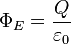# Electric field at a radius outside of a hollow cylinder w/ a rod in it

PhizKid

## Homework Statement

Consider a hollow cylinder of radius 10 m., length 50 m., and charge 6 C., and a rod with radius 4 m., length 50 m., and charge -4 C. The rod is uniformly placed inside the center of the hollow cylinder. What is the electric field at radius = 15 m.?

## Homework Equations## The Attempt at a Solution

I am unsure where to place my Gaussian surface(s). Do I need more than one? Does the space between the cylinder and rod matter? It seems like the electric field outside of the cylinder will be independent of the rod's radius. It sounds like a trick question though, because the problem gave the radius of the rod and extraneous information is never given for these problems, although I might be wrong about this.

Last edited by a moderator:

Homework Helper
Lynchpin: the electric field due to two objects is the sum of the fields due to each one by itself.

To answer your question: your Gaussian surface is dictated by the geometry. You only ever need the one.

PhizKid
Sorry, I didn't mean to ask if I needed more than one geometry. I meant to ask if I need to use that geometry more than once.

But Gauss' Law says that the flux is equal to the total charge enclosed over the epsilon thing. If I choose my Gaussian cylinder to enclose the entire cylinder+rod, is that a valid thing to do? Or do I have to calculate the field due to the rod and add it to the field due to the cylinder around it using two or more Gaussian cylinders?

Gold Member
2021 Award
Hm, I think the problem is not completely stated. The question is, where you should calculate the field. They give only "the radius" but not where along the cylinder axis it's located. At least the outer cylinder is not so much longer than its radius, so that a complete cylinder symmetric approximation might not be sufficient. I've not checked this, however.

Homework Helper
If I choose my Gaussian cylinder to enclose the entire cylinder+rod, is that a valid thing to do?
Yep.

Or do I have to calculate the field due to the rod and add it to the field due to the cylinder around it using two or more Gaussian cylinders?
Do it both ways and see :) Does it matter?

Have you not already calculated solutions for a rod and for a cylinder - perhaps in another problem, or in class?

Note: vanhees71 is correct - I suspect that the way you wrote out the question is not the way it is presented to you (or the question assumes stuff - like that the field is to be calculated equal distance from the end-points.)

Homework Helper
Gold Member
Sorry, but I feel the need to jump in here.

The problem statement is poorly worded. For one, it doesn't specify the nature of the charge distribution over the rod and cylinder.

We can assume that the respective charges are uniformly distributed along the lengths of the rod and cylinder, but that is an assumption on our part: something the original problem statement should have specified.

We can assume that the problem statement is asking for the electric field 15 meters above the centers of the rod/cylinder combination. But the problem statement does not specify above the center. That would be another assumption made on our part.

"The rod is uniformly placed inside the center of the hollow cylinder." What the heck does that mean? As opposed to non uniformly? I don't even know what that's trying to say.

It is not possible to use Gauss' law to to precisely find the electric field for this problem. Gauss's law still holds of course, it's just not possible to use it to find the electric field unless certain conditions are met. For situations involving cylindrical charge distribution, the cylinders/rods must be infinitely long in length if you want to use Gauss' law to find the electric field. If you really want to find the electric field for this problem precisely, you'll have to use integration.

That said, it is possible to approximate the electric field using Gauss' law, and using all the assumptions that we made such as uniform charge distribution, electric field 15 m above the center of the rod/cylinder combination, etc. It's a valid approximation if the height above the center of charge is much, much smaller than the length. (Is 15 m << 50 m? That's kind of a stretch if you ask me.) It would have been nice if the problem statement said to approximate the electric field using Gauss' law, but it didn't really say that. So that's one more assumption on our part.

So PhizKid, back to your original question. If you are going to use Gauss' law to approximate the electric field at 15 meters above the center of the rod/cylinder combination, and all our other assumptions are valid, then your Gaussian surface needs to be thin disk-like cylinder, 15 meters in radius, but very thin. The Gaussian surface cylinder should share the same axis and center as the charged cylinder and charged rod. Assume that all electric field lines exit the Gaussian cylinder at the thin strip at its perimeter, and no electric field lines exit the Gaussian surface through its large, disk-shaped sides.

[Edit: this Gaussian surface that I described is equivalent to making a different assumption that the 50 m rod and cylinder is just part of a much larger coaxial, charged rod and cylinder. In both cases, the important part of the approximation is to assume that all electric field lines go through the curved part of the Gaussian surface cylinder, and no electric field lines go through the circular caps.]

Last edited: